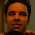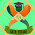## Thursday, 9 February 2012

### GARCH estimation using maximum likelihood

In my previous post I presented my findings from my finance project under the guidance of Dr Susan Thomas. The results in my paper suggested that there are macroeconomic variables, particularly the INR/USD exchange rates, that help us understand the dynamics of stock returns. Although the results that I obtained were significant at 5% level, the weight of my assertion needs some more robust check before we consider the matter closed. One possible source of discrepancy we identified was that the error terms could be heteroskedastic. Meaning that one of our assumption of classical linear regression model (CLRM) estimation viz. homoskedasticity is violated. The resultant coefficient estimated, in case of heteroskedasticity of the error terms, can have underestimated standard errors, which in turn might lead to false acceptance/rejection of our null hypothesis. There are several parametric as well as non-parametric ways to remove the effect of heteroskedasticity in the error terms on the coefficient estimates. However, the method that I adopted to correct for this effect in my model was the path breaking conditional heteroskedasticity modelling propagated by Robert Engle for which he was awarded the Nobel prize in 2003.

The idea behind auto regressive conditional heteroskedasticity (ARCH) model is quite simple and straightforward. One needs to model the mean equation (this is your regression model) and along with that there has to be a specification for the modelling the squares of the errors. Suppose we have a simple AR regression:

zt = φ*zt-1 + et        (Mean equation)

Now to model the conditional variance of the error terms all we need to do is a simultaneous estimation of the squares of the error terms in the following manner:

et = ѵtht1/2 ,  where ѵt  ~ NID(0,1)
ht = α0 + α1et-12      (Variance equation)

The above specification of the mean and the variance equations is termed as a AR(1) ARCH(1) specification. This simultaneous estimation takes into account the particular form of heteroskedasticity (ARCH(1) in this case) and estimates the 'φ' coefficient accordingly. Now the above simple specification tends to pose another problem of lag selection, what lag should be considered for the variance equation. To deal with this and several other shortcomings of the simple ARCH model, Bollerslev(1986)  proposed a generalized ARCH model (GARCH). The only difference being that the variance equation now becomes:

ht = α0 + α1et-12 + βht-1

Which is nothing but a GARCH(1,1) model. The beauty of this specification is that a GARCH(1,1) model can be expressed as an ARCH(∞) model. For those who are interested in learning more about ARCH and GARCH processes and the mathematics behind them here are Dr Krishnan's notes that provide an in-depth understanding on the matter. The reason why the ARCH and GARCH models rose to such prominence was because they offered us a method of not just correcting the standard errors of the estimates (as like other robust correction methods) but also provided a functional form for modelling the variance. This idea of modelling variance was heavily adopted by financial researchers. Considering the standard deviation (or variance) of asset prices as a crude estimate of volatility, financial researchers used ARCH and GARCH models to model asset price and/or other high frequency index volatility.

Now that the idea behind the GARCH modelling is clear let us plunge right into writing the conditional maximum likelihood function for a GARCH process.

Utkarsh was the generous one who provided me with the basic structure of the codes that I then customized to solve this problem at hand. Well now let me confess that a similar result or in fact a more accurate estimates of the coefficients can be obtained using unconditional maximum likelihood estimates that are offered by any of the high priced computer packages like EVIEWS, RATS, SAS etc but my motivation (apart from learning ofcourse) was to figure out a way that could give me the flexibility of modelling any functional form of heteroskedasticity. The above codes have been presented for the estimation of a GARCH(1,1) model but I could do a simple manipulation in the definition of hto fit any arbitrary functional form.

P.S Sincere thanks to Utkarsh for letting me share the codes. Any feedback/suggestions are welcome.

1.Hi, sorry to bother~
I need to add some other error terms to GARCH(1,1),
the function is like this:
h_t=a_0+a_1*(e_(t-1))^2+b*h_(t-1)+a_2(e_(t-1))'^2
the second e is from another data
what should I do in R to do the estimation?
I am so confused...

1.Hi Du Yuchen,

I think what you are saying here is that you have a multivariate timeseries regression, where you have z1t and z2t, which give you two different error terms(e1t and e2t). In this case you should go for a MGARCH(multivariate-GARCH) and not a simple GARCH.

The likelihood estimation of a MGARCH is quite difficult to code(at least for me). You could look into packages like "mgarch", "mgarchBekk", "ccgarch", and "gogarch" which could help you get this done in a simple function.

~
Shreyes

2.I know the data I use is a multivariate one but is the thought that I only use a univariate model possible?

3.No you cannot use the univariate GARCH model here since you have 2 conditional variance to model(var(e1t) and var(e2t)). You will have to have at least 2 equations for them(h_1t and h_2t).

Infact matter doesnt end here, now that you have 2 et's you will also have to worry about the cross correlations(h_12t). All these can be easily dont in the BEKK model, refer to the packages in my earlier reply.

Hope this helps.

~
Shreyes

4.Ok, I got it.
Thanks very much:)

2.Please help me with the code i have. Though the code converges, the issue is that for different initial values , it converges to a different value. Though these values are close to each other, but still this would mean that the code is not stable. Also i am unable to get the Hessian. Kindly point out the error in the code. I will be extremely grateful.

s_estimate <- function(param)

{
phi0 <- param
phi1 <- param
omega <- param
alpha1 <- param
beta1 <- param
mean_error1 <- mean_error
sigma_square1 <- sigma_square

for ( j in 2:l_USA)
{
mean_error1[j] <- (Returns_USA[j]-phi0-phi1*Returns_USA[j-1])
sigma_square1[j] <-(omega+alpha1*((mean_error1[j-1])^2)+beta1*sigma_square1[j-1])
}

term1 <- (log(2*pi*sigma_square1))
#print(term1)
term2 <- (mean_error1)^2/(2*sigma_square1)
term3 <- (term1+term2)
val <- (-1)*sum((-0.5)*term3)
return(val)
}

The likelihood is for AR(1)-GARCH(1,1) model.

3.Is there in one of the packages inherent the VECH model estimation?

4.Thanks ya, artikel sangat membantu dalam menyelesaikan tugas perkuliahan tentang Generalized AutoRegressive Conditional Heteroskedastisitas (GARCH). Kunjungi juga ya MAKALAH GARCH

5.